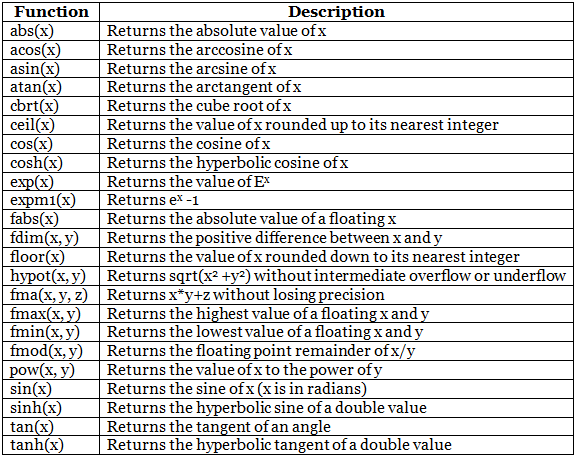C++ Math & Booleans

# C++ Math & Booleans - Notes | Study C++ Programming for Beginners - Class 8

 1 Crore+ students have signed up on EduRev. Have you?

C++ Math

C++ has many functions that allows you to perform mathematical tasks on numbers.

Max and min

The max(x,y) function can be used to find the highest value of x and y:

Example

cout << max(5, 10);

And the min(x,y) function can be used to find the lowest value of x and y:

Example

cout << min(5, 10);

Other functions, such as sqrt (square root), round (rounds a number) and log (natural logarithm), can be found in the <cmath> header file:
Example

// Include the cmath library

#include <cmath>

cout << sqrt(64);

cout << round(2.6);

cout << log(2);

Other Math Functions

A list of other popular Math functions (from the <cmath> library) can be found in the table below:C++ Booleans

Very often, in programming, you will need a data type that can only have one of two values, like:

• YES / NO
• ON / OFF
• TRUE / FALSE

For this, C++ has a bool data type, which can take the values true (1) or false (0).

Boolean Values

A boolean variable is declared with the bool keyword and can only take the values true or false:

Example

bool isCodingFun = true;

bool isFishTasty = false;

cout << isCodingFun;  // Outputs 1 (true)

cout << isFishTasty;  // Outputs 0 (false)

From the example above, you can read that a true value returns 1, and false returns 0.
However, it is more common to return boolean values from boolean expressions

C++ Boolean Expressions

A Boolean expression is a C++ expression that returns a boolean value: 1 (true) or 0 (false).
You can use a comparison operator, such as the greater than (>) operator to find out if an expression (or a variable) is true:
Example

int x = 10;

int y = 9;

cout << (x > y); // returns 1 (true), because 10 is higher than 9

Or even easier:

Example

cout << (10 > 9); // returns 1 (true), because 10 is higher than 9

In the examples below, we use the equal to (==) operator to evaluate an expression:

Example

int x = 10;

cout << (x == 10);  // returns 1 (true), because the value of x is equal to 10

Example

cout << (10 == 15);  // returns 0 (false), because 10 is not equal to 15

The document C++ Math & Booleans - Notes | Study C++ Programming for Beginners - Class 8 is a part of the Class 8 Course C++ Programming for Beginners.
All you need of Class 8 at this link: Class 8

## C++ Programming for Beginners

15 videos|20 docs|13 tests
 Use Code STAYHOME200 and get INR 200 additional OFF

## C++ Programming for Beginners

15 videos|20 docs|13 tests

Track your progress, build streaks, highlight & save important lessons and more!

,

,

,

,

,

,

,

,

,

,

,

,

,

,

,

,

,

,

,

,

,

;